## 5.1 Graphical Methods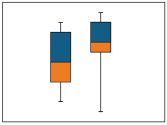Graphs are powerful data evaluation tools. They provide quick, visual summaries of essential data characteristics. A few simple plots can replace complex statistical equations or tests to interpret environmental data. Box plots, histograms, and normal probability plots are examples of graphs that are commonly used to display environmental data. These graphs can provide information about concentration ranges, shapes of distributions, extreme values (outliers), relationships between different data sets, and trends (increasing, decreasing, and cyclic). Because graphical methods are qualitative, however, they may not be appropriate as a stand-alone technique to make inferences or support conclusions.

Graphical methods are typically used with quantitative statistical evaluations. Graphical methods provide information that may not be otherwise apparent from quantitative statistical evaluations, so it is a good practice to evaluate data using these methods prior to performing statistical evaluations. Graphical methods are also a key component of exploratory data analysis (EDA). In EDA, various graphical techniques are used initially to display data for qualitative assessments prior to selecting appropriate statistical tests. Brief descriptions of some useful statistical plots are presented in the subsections below.

### 5.1.1 Time Series Methods

Time series methods graph data of interest, such as concentration, on the y-axis versus time on the x-axis. When plotting multiple series, it may be helpful to standardize or normalize data prior to plotting. Time series plots include lag-plots, correlogramsA plot of the autocorrelation coefficients versus the time lags. This plot is also known as an autocorrelation plot., and variograms.

Lag-plots. Lag plots display observations for a time series against a later set of observations, or against the difference between the two (for example, a plot of x(t) versus x(t-1). If the lag plotA plot that displays observations for a time series against a later set of observations, or against the difference between the two sets. exhibits a linear pattern, it follows that data are nonrandom and that you may need to use an autoregressive model. If no patterns are discernible in the lag plot, data are likely random. Plotting data for a greater number of observational periods or lags can be helpful in evaluating data for seasonality. An example of a lag plot is provided in Figure 5-1.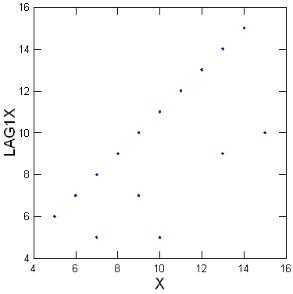Figure 5-1. Lag plot example.

Correlograms. Correlograms are commonly used to evaluate the randomness in a data set. Correlograms (or, autocorrelationCorrelation of values of a single variable data set over successive time intervals (Unified Guidance). The degree of statistical correlation either (1) between observations when considered as a series collected over time from a fixed sampling point (temporal autocorrelation) or (2) within a collection of sampling points when considered as a function of distance between distinct locations (spatial autocorrelation). plots) display the correlationAn estimate of the degree to which two sets of variables vary together, with no distinction between dependent and independent variables (USEPA 2013b). between two variables (for example, a plot of the autocorrelation function versus the lag) and provide a graphical evaluation of temporal dependence. Autocorrelations may be calculated for data values at varying time lags. If the data are random, the autocorrelation value should be near zero for all time lags (i.e., the autocorrelation plot at time x+1 should not be significantly different than the plot for time x+2, and so forth). A sample correlogram displaying nonrandom data are provided as Figure 5-2.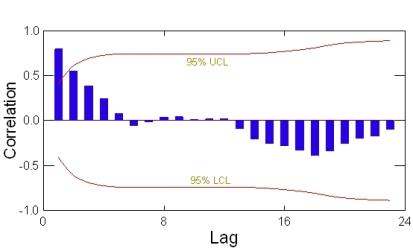Figure 5-2. Correlogram example.

Variograms. Variograms (also known as a semi variogramA plot of the variance (one-half the mean squared difference) of paired sample measurements as a function of the distance (and optionally the direction) between samples. Typically, all possible sample pairs are examined, distance and directions. Variograms provide a means of quantifying the commonly observed relationship that samples close together will tend to have more similar values than samples far apart (EPA 1989). A graphical tool used in geostatistical analysis.) plot a variogram coefficient associated with a selected model of temporal or spatial correlation versus data from different lags and angles in an effort to fit the selected model to the data. The selected model is subsequently used in krigingA weighted moving-average technique to interpolate the data distribution by calculating an area mean at nodes of a grid (Gilbert 1987). for contouring of the data. An example of a variogram is provided in Figure 5-3.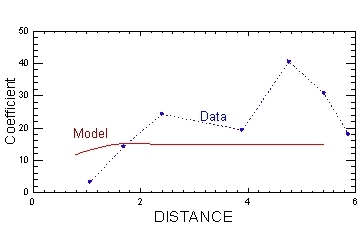Figure 5-3. Variogram example.

Time series plots show the following:

• concentration trends over time
• lack of randomness
• changes in location (for example, of a plume or of the highest concentrations)
• degradation (when concentration vs. time plots are viewed for a contaminant and its degradation by-products)

Figure 5-4 illustrates a time series plotA graphic of data collected at regular time intervals, where measured values are indicated on one axis and time indicated on the other. This method is a typical exploratory data analysis technique to evaluate temporal, directional, or stationarity aspects of data (Unified Guidance). with data from two monitoring wells over seven years.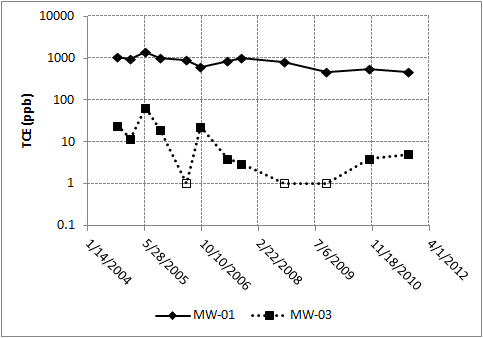Figure 5-4. Time series plot example.

### 5.1.2 Box Plots

Box plots divide data into four groupings, each of which contain 25% of the data. The box most typically depicts the 25th (bottom of the box), 50th (horizontal line within the box) and 75th (top of box) percentile values while the whiskers can be selected to represent various extremes such as 1.5 times the interquartile rangeThe middle range of an ordered set of sample values between the 25th and 75th sample percentiles (Unified Guidance). (Tukey 1977), or 0% and 100% values. Points falling outside of the range depicted by the whiskers are plotted as individual points; you can evaluate these points as potential outliers. The meanThe arithmetic average of a sample set that estimates the middle of a statistical distribution (Unified Guidance). and the 95% upper confidence limit (UCL)The upper value on a range of values around the statistic (for example, mean) where the population statistic (for example, mean) is expected to be located with a given level of certainty, such as 95% (science-dictionary.org 2013). and lower confidence limit (LCL)The lower value on a range of values around the statistic (for example, mean) where the population statistic (for example, mean) is expected to be located with a given level of certainty (science-dictionary.org 2013). are often depicted on a box plotGraphic of selected descriptive statistics at a monitoring point such as mean, median, or upper and lower quartiles (Unified Guidance). as well.

The extent of the box is the interquartile range, which is the range of values between the 25th and 75th percentiles. A common convention is for whiskers to extend to 1.5 times the interquartile range on either side of the box. In this case, values between 1.5 and 3 times the interquartile range outside the whiskers are typically considered “mild” outliersValues unusually discrepant from the rest of a series of observations (Unified Guidance). while values greater or less than 3 times the interquartile range are considered “extreme” outliers. Graphing two data sets on side-by-side box plots provides an easy method of data comparison.

Figure 5-5 illustrates a box plot.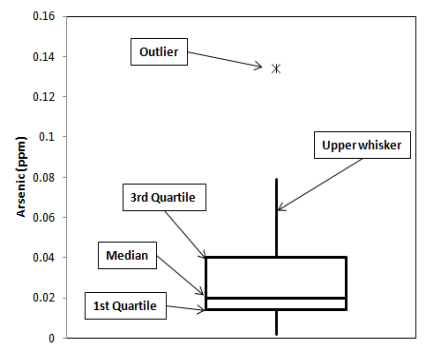Figure 5-5. Box plot example

### 5.1.3 Scatter Plots

Scatter plots display the relationship between two or three variables when comparing data sets consisting of multiple observations per sampling pointA specific spatial location from which groundwater is being sampled.. Linear relationships will manifest in points clustering about a straight line. Figure 5-6 illustrates a scatter plot.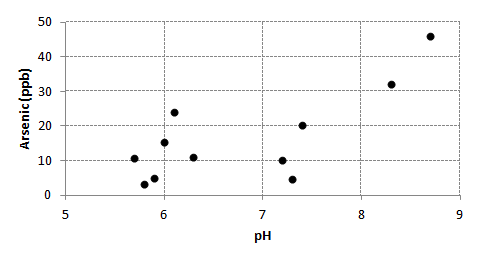Figure 5-6. Scatter plot example.

### 5.1.4 Histograms

Histograms present data in terms of bars of height (Y) in relation to a parameter (X), permitting a comparison of the shape and size of the plot, and of the placement of the plot along the x-axis.

Figures 5-7 illustrates a bimodal distributionA data distribution that has two peaks or two modes (science-dictionary.org 2013; NIST/SEMATECH 2012). of data in a histogram.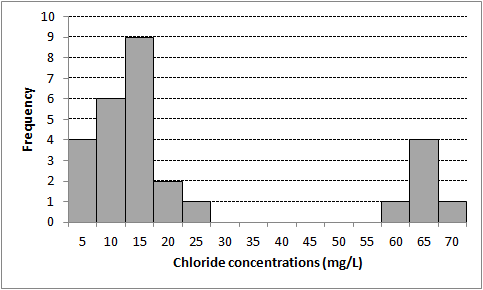Figure 5-7. Histogram example (bimodal distribution).

Figure 5-8 illustrates a non-normal and skewed distribution of data in a histogram.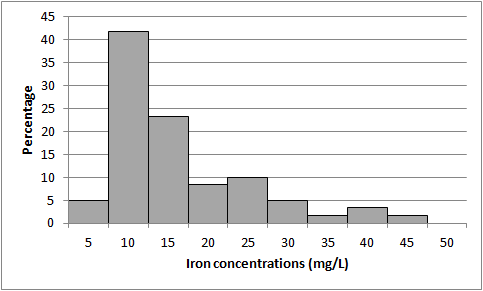Figure 5-8. Histogram example (non-normal and skewed distribution).

### 5.1.5 Probability Plots

Probability plots help to evaluate how well data fit a theoretical distribution, such as a normal distributionSymmetric distribution of data (bell-shaped curve), the most common distribution assumption in statistical analysis (Unified Guidance)., or gammaA gamma distribution or data set. A parametric unimodal distribution model commonly applied to groundwater data where the data set is left skewed and tied to zero. Very similar to Weibull and lognormal distributions; differences are in their tail behavior, and the gamma density has the second longest tail where its coefficient of variation is less than 1 (Unified Guidance; Gilbert 1987; Silva and Lisboa 2007). distribution. Probability plots express the theoretical distribution as a straight line and departures from the distribution appear as departures from the straight line. Data skewness or asymmetry, presence of outliers, and heavy tails of the data distribution (non-normal distribution) are obvious on probability plotsGraphical presentation of quantiles or z-scores plotted on the y-axis and, for example, concentration measurement in increasing magnitude plotted on the x-axis. A typical exploratory data analysis tool to identify departures from normality, outliers and skewness (Unified Guidance).. If the data do not fit the selected distribution, data can be transformed using a lognormalA dataset that is not normally distributed (symmetric bell-shaped curve) but that can be transformed using a natural logarithm so that the data set can be evaluated using a normal-theory test (Unified Guidance). or other transformation in order to determine whether data fits an alternative distribution. A quantile-quantile plotA graph of the ranked data versus the fraction of data points it exceeds (USEPA 2006c). may be used to compare two empirical distributions.

To generate probability plots, order the data, and calculate matching percentiles from the normal distribution. Plot the ordered data against the percentiles and examine the plot for a straight-line fit. The straightness of the plot indicates how closely the data fit a normal distribution. If all of the raw data closely follow a straight line, the suspected outliers are probably part of the same distribution and should not be considered outliers. Points that appear off of a linear pattern in the rest of the data may be outliers; however, be aware that other reasons, such as non-normal data, can also explain nonlinearity.

Figure 5-9 illustrates a data set as a probability plot. Figure 5-10 presents the same data in a histogram. Figure 5-11 presents the logarithms of the same data as a probability plot and Figure 5-12 presents the histogram of the log transformed data.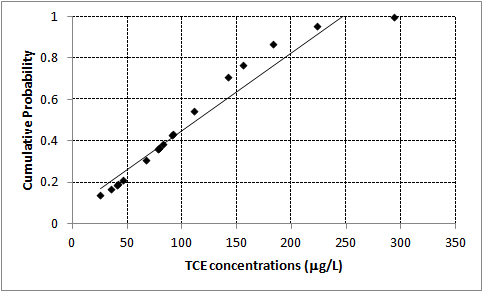Figure 5-9. Data set as a probability plot.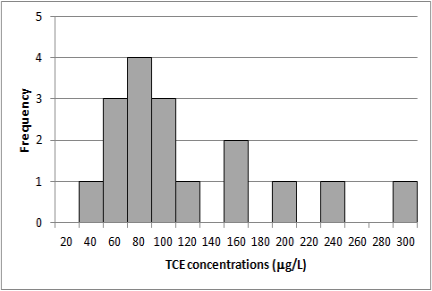Figure 5-10. Data set as a histogram.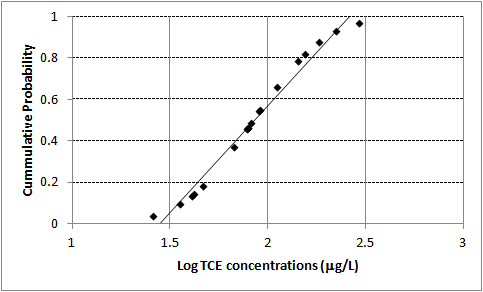Figure 5-11. Logarithms of data set as a probability plot.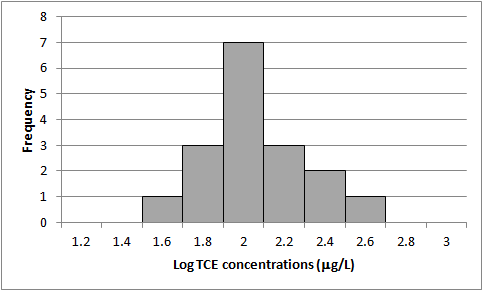Figure 5-12. Histogram of log-transformed data.

Publication Date: December 2013

Permission is granted to refer to or quote from this publication with the customary acknowledgment of the source (see suggested citation and disclaimer).

This web site is owned by ITRC.

1250 H Street, NW • Suite 850 • Washington, DC 20005

(202) 266-4933 • Email: [email protected]

ITRC is sponsored by the Environmental Council of the States.